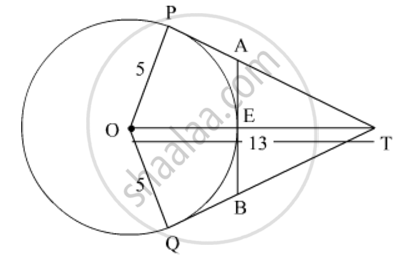# In Fig. 8, O is the centre of a circle of radius 5 cm. T is a point such that OT = 13 cm and OT intersects circle at E. If AB is a tangent to the circle at E, find the length of AB, where TP and TQ are two tangents to the circle. - Mathematics

In Fig. 8, O is the centre of a circle of radius 5 cm. T is a point such that OT = 13 cm and OT intersects circle at E. If AB is a tangent to the circle at E, find the length of AB, where TP and TQ are two tangents to the circle.#### Solution

From the given figure, we have

TP = TQ                              (Two tangents, drawn from an external point to a circle, have equal length.)

and

TQO=TPO=90°                 (Tangent to a circle is perpendicular to the radius through the point of contact.)

In ∆TOQ,

QT2+OQ2=OT2

QT2=13252=144

QT=12 cm

Now,

OT − OE = ET = 13 − 5 = 8 cm

Let QB = x cm.

∴ QB = EB = x      (Two tangents, drawn from an external point to a circle, have equal length.)

Also,

OEB = 90°        (Tangent to a circle is perpendicular to the radius through the point of contact.)

In ∆TEB,

EB2+ET2=TB2

x2+82=(12x)2

x2+64=144+x224x

24x=80

=>x = 80/24=10/3

:.AB=2x=20/3cm

Thus, the length of AB is 20/3 cm

Concept: Concept of Circle - Centre, Radius, Diameter, Arc, Sector, Chord, Segment, Semicircle, Circumference, Interior and Exterior, Concentric Circles
Is there an error in this question or solution?
2015-2016 (March) Delhi Set 1
Share